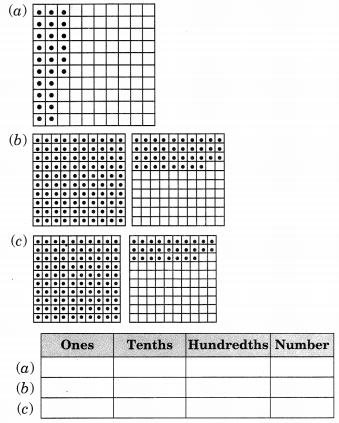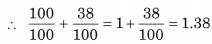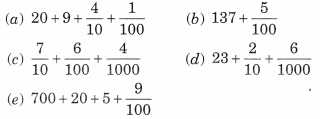# NCERT Solutions For Class 6 Maths Decimals Exercise 8.2

ncert textbook

## NCERT Solutions For Class 6 Maths Decimals Exercise 8.2

NCERT Solutions for Class 6 Maths Chapter 8 Decimals Ex 8.2

Exercise 8.2

Question 1.
Complete the table with the help of these boxes and use decimals to write the number.Solution:

 Ones Tenths Hundredths Number (a) 0 2 6 0.26 (b) 1 3 8 1.38 (c) 1 2 8 1.28

Explanation:
(a) In this figure,
If small block out of 100 are shaded
∴ Decimal representation = 0.26

(b ) 100 small blocks + 38 small blocks are shaded∴ Decimal representation = 1.38

(c) 100 small blocks + 28 small blocks are shaded∴ Decimal representation = 1.28

Question 2.
Write the numbers given in the following place value table in decimal form:Solution:
(a) 0 Hundreds + 0 Tens + 3 Ones + 2 Tenths + 5 Hundredths + 0 Thousandths= 3 + 0.2 + 0.05 + 0.000 = 3.250 = 3.25

(b) 1 Hundreds + 0 Tens + 2 Ones + 6 Tenths + 3 Hundredths + 0 Thousandths= 102.630 = 102.63

(c) 0 Hundreds + 3 Tens + 0 Ones + 0 Tenths + 2 Hundredths + 5 Thousandths
= 0 x 100 + 3 x 10 + 0 x 1 + 0 x$\frac { 1 }{ 10 }$+ 2 x$\frac { 1 }{ 100 }$+ 5 x$\frac { 1 }{ 1000 }$
= 0 + 30 + 0 + 0.0 + 0.02 + 0.005
= 30.025

(d) 2 Hundreds + 1 Tens + 1 Ones + 9 Tenths + 0 Hundredths + 2 Thousandths
= 2 x 100 + 1 x 10 + 2 x$\frac { 1 }{ 10 }$+ 0 x$\frac { 1 }{ 100 }$+ 2 x$\frac { 1 }{ 100 }$
= 200 + 10 + 1 + 0.9 + 0.00 + 0.002
= 211 + 0.902 = 211.902

(e) 0 Hundreds + 1 Tens + 2 Ones + 2 Tenths + 4 Hundredths + 1 Thousandths
= 0 x 100 + 1 x 10 + 2 x 1 + 2 x$\frac { 1 }{ 10 }$+ 4 x$\frac { 1 }{ 100 }$+ 1 x$\frac { 1 }{ 1000 }$
= 0 + 10 + 2 +$\frac { 2 }{ 10 }$+$\frac { 4 }{ 100 }$+$\frac { 1 }{ 1000}$
= 12 + 0.2 + 0.04 + 0.001 = 12.241

Question 3.
Write the following decimals in the place value table.
(a) 0.29
(b) 2.08
(c) 19.60
(d) 148.32
(e) 200.812
Solution:
(a) 0.29 = 0 + 0.2 + 0.09 = 0 Ones + 2 Tenths + 9 Hundredths
(b) 2.08 = 2 + 0.0 + 0.08
= 2 Ones + 0 Tenths + 8 Hundredths
(c) 19.60 = 10 + 9 + 0.6 + 0.00
= 1 Tens + 9 Ones + 6 Tenths + 0 Hundredths
(d) 148.32 = 100 + 40 + 8 + 0.3 + 0.02
= 1 Hundred + 4 Tens + 8 Ones + 3 Tenths + 2 Hundredths
(e) 200.812 = 200 + 0.8 + 0.01 + 0.002
= 2 Hundreds + 8 Tenths + 1 Hundredth + 2 Thousandths
The above information, we can gives in place value Table:

 Hund­reds (100) Tens (10) Ones (1) Ten­ths (1/10) Hund­redths (1/100) Thous­andths (1/1000) (a) 0 0 0 2 9 0 (b) 0 0 2 0 8 0 (c) 0 1 9 6 0 0 (d) 1 4 8 3 2 0 (e) 2 0 0 8 1 2

Question 4.
Write each of the following as decimals.Solution:Question 5.
Write each of the following decimals in words.
(a) 0.03
(b) 1.20
(c) 108.56
(d) 10.07
(e) 0.032
(f) 5.008
Solution:
(a) 0.03 = Zero point zero three
(b) 1.20 = One point two zero
(c) 108.56 = One hundred eight point fifty-six
(d) 10.07 = Ten point zero seven
(e) 0.032 = Zero point zero three two
(f) 5.008 = Five point zero zero eight

Question 6.
Between which two numbers in tenths place on the number line does each of the given numbers lie?
(a) 0.06
(b) 0.45
(c) 0.19
(d) 0.66
(e) 0.92
(f) 0.57
Solution:
(a) 0.06 lies between 0 and 0.1
(b) 0.45 lies between 0.4 and 0.5
(c) 0.19 lies between 0.1 and 0.2
(d) 0.66 lies between 0.6 and 0.7
(e) 0.92 lies between 0.9 and 1.0
(f) 0.57 lies between 0.5 and 0.6

Question 7.
Write as fraction in lowest terms.
(a) 0.60
(b) 0.05
(c) 0.75
(e) 0.25
(f) 0 .125
(g) 0.066